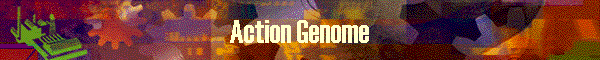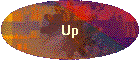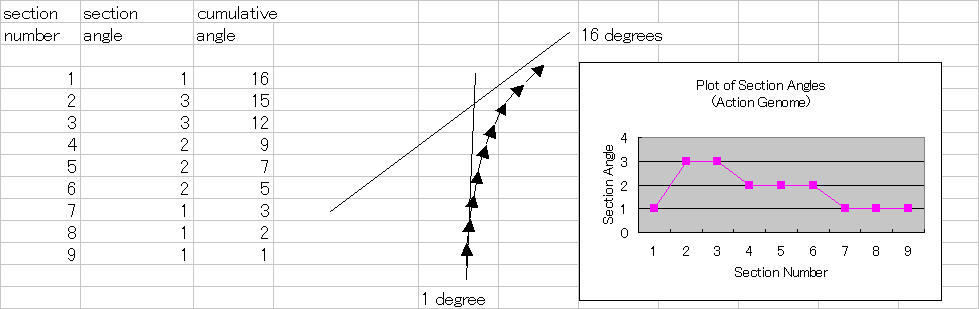This graph shows the plot of bend angle of each 1" section of the rod.

Bend angle is the angle to the previous section.  Physically, Rod Deflection consists of  the accumulation of bend angle of each 1" section from butt to tip top.

We can use this graph when we design bend pattern of a rod as we did on  statically calculated stress curve before.    Bend pattern is related to the action of the rod very much.

There are several kind of patters such as progressive, flat and parabolic type action.

### What is Action Genome?

In Deflection Designer Excel Tool, there is an explanation of Rod Deflection and Action Genome.  The following is the extracts from the tools explanation;

The user can understand how a rod would deflect and why it deflects that way through visible graphic presentation.

Prior to start using this tool, users should understand that a rod deflection consists of the aggregate of bending angles of each subdivided sections of a rod. The following picture shows this relationship very well, where a rod is subdivided into 1" sections. Angles and tangent lines are measured at the center line of a rod.[UP] in navigation bar at left will show you another explanation of Rod Deflection and Action Genome.

Genome graph shows two measurement lines.  One is the line of 1 degree line.
The other is the averaged bend angle line calculated via  (Top Angle) divided by (Action Length)

These lines does not have specific meanings but the average line can be compared to 1 degree line
so that we can know how much the average line is apart from 1 degree line, upper side or lower side, etc..
I hope this kind of measurement would generate a new rules of thumb measurement.

On Genome graph, it may rather be important that which part of the rod section is above 1 degree line
and where the part is located along with the rod.   If the tip part is above 1 degree line (or average angle line),
it says that the rod bend much at tip part, that is tip action type.  Like that.